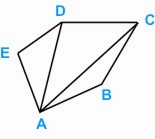Question: What is a Diagonal ? Answer: A diagonal is a straight line from a vertex of a polygon to any other vertex of the polygon not next to it. For example,There are two diagonals from A in the pentagon namely AC and AD. The word diagonal derives from the Greek, later adopted into Latin as diagonus (slanting line). In mathematics, in addition to its geometric meaning, a diagonal is also used in matrices to refer to a set of entries along a diagonal line.